# 5 Nonlinear Wave Structures

To consider the nonlinear features of electrostatic wave structures, we have numerically solved Eq. (36) for various plasma parameters, in order to investigate their effects. We found that both negative and positive electric potentials arise in the ranges of allowed Mach numbers obtained for negative and positive polarity soliton existence domains in Section 4.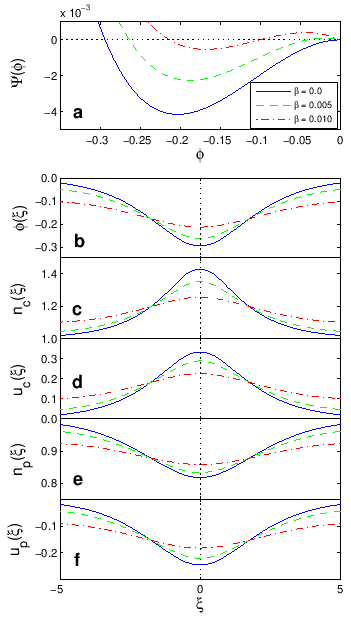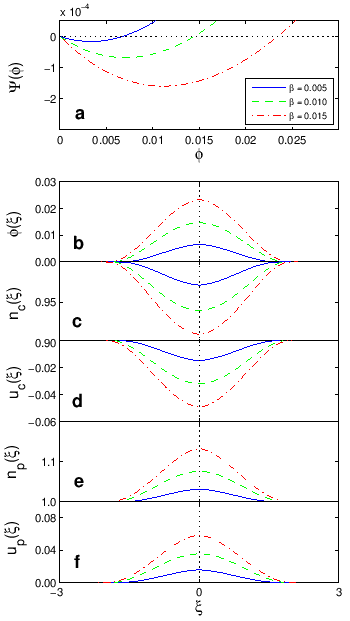Figure 4(a) shows the variation of the pseudopotential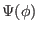of negative polarity solitons with the normalized negative potential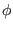, for different values of the positron-to-cool electron density ratio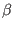(keeping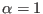,,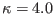and Mach number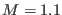, all fixed). The electrostatic pulseshown in Fig. 4(b) is obtained via a numerical integration. The negative pulse amplitude decreases with increasing. We algebraically determined the fluid density (Fig. 4c) and velocity disturbance (Fig. 4d) of the cool electrons, as well as the fluid density (Fig. 4e) and velocity disturbance (Fig. 4f) of the positrons. It is found that an increase in the positron-to-cool electron density ratiodecreases the disturbances and amplitudes of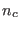,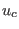,andin the negative polarity electrostatic mode. This means that increasing the positron density reduces the negative potential solitary waves, in agreement with the previous results . We also note that the profiles become less steeper but broader.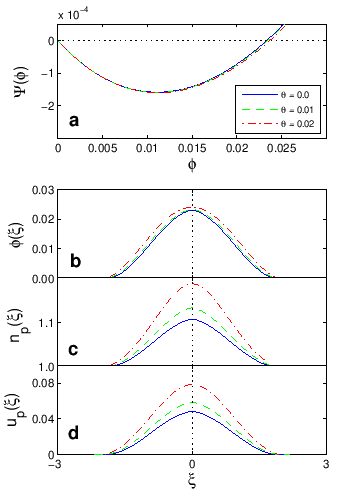Similarly, Figure 5(a) depicts the variation of the pseudopotentialof positive polarity solitons associated with the positrons for different values of the positron-to-cool electron density ratio(keeping,,and Mach number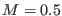, all fixed). As seen in Fig. 5(b), that the positive pulse amplitude rises with an increase in, in contrast to what we see in Fig. 4(b). Furthermore, an increase inincreases the disturbances, amplitudes and steepness of,andin the positive polarity electrostatic mode. This means that increasing the positron density increases the positive potential solitary waves, which agrees with the results of Ref.  (they used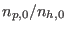rather than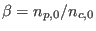).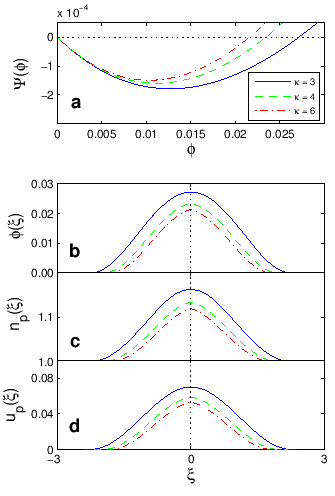The thermal effect of the positrons through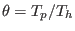is shown in Fig. 6. The soliton excitationis slightly amplified with an increase in the temperature ratio, which agrees with the results of Ref.  (they usedrather than). Furthermore, an increase inslightly increases the disturbance ofand(not shown here), however, significantly increases and steepens the disturbance ofandin the positive polarity electrostatic mode (Fig. 6(c) and (d)). The temperature ratiodoes not make a significant contribution to the negative polarity electrostatic solitary waves due to the small value of.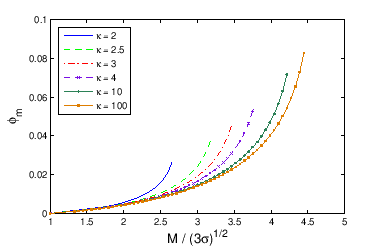Figure 7(a) shows the pseudopotentialof positive polarity solitons for different values of the spectral index(keeping,,and Mach number). The positive polarity electrostatic pulse shown in Fig. 7(b) is found to increase for lower, implying an amplification of the electric potential disturbance as the suprathermality increases. It can be seen that the positron fluid density (Fig. 7(c)) and velocity disturbance (Fig. 7(d)) are increased in the positive polarity electrostatic mode, and again, for lowervalues.

As inherently super-acoustic solitons are taken, it is important to see the effect of a varying true Mach number, so we explore the pulse amplitudeof the positive polarity electrostatic solitons as a function of the propagation speed, measured relative to the true acoustic speed,. The variation of the soliton amplitudeas a function of the true Mach number,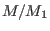, is numerically obtained from Eq. (36). Noting that the lower limit (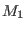) for positive polarity solitary structures is about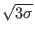, we have plotted the soliton amplitude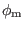against the ratio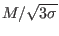, for a range of values of the parameterin Fig. 8. It is seen that the soliton amplitudeincreases withfor all values of. Moreover, the soliton amplitude increases with growing the suprathermality (reducing) at a fixed true Mach number,, in contrast to the results obtained previously . However, the maximum value of soliton amplitude is found to be for a Maxwellian distribution (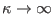) at larger true Mach numbers ().

Ashkbiz Danehkar
2018-03-28